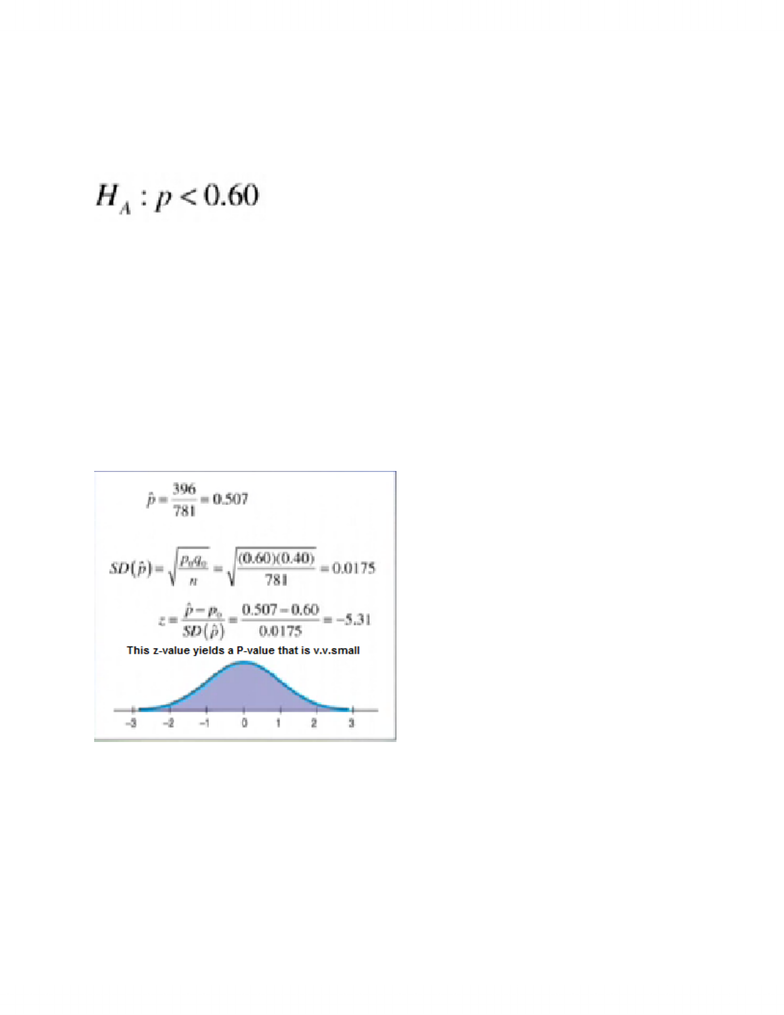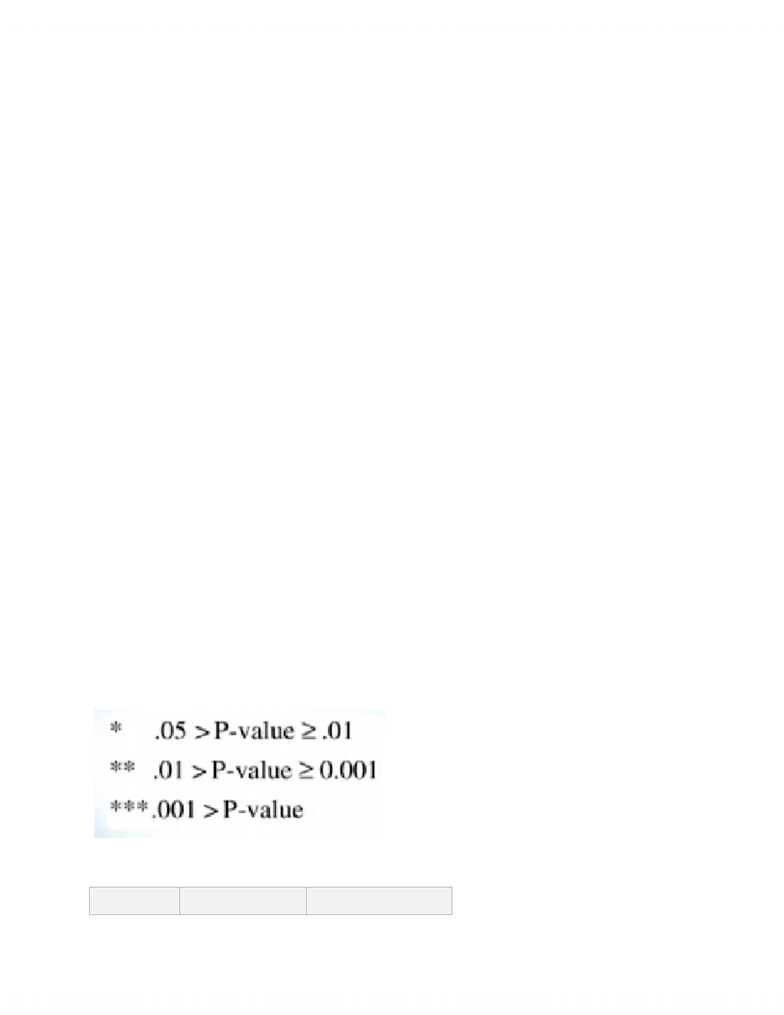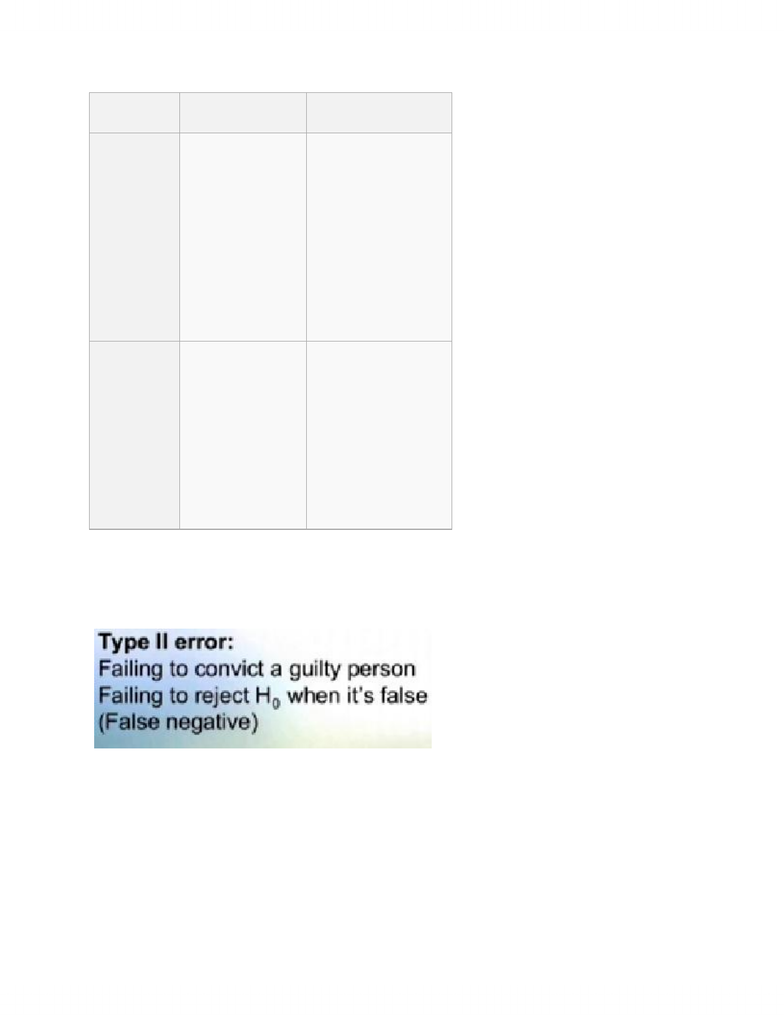# STAB22H3 Chapter Notes -Type I And Type Ii Errors, Null Hypothesis, Statistic

21 views45 pages
School
Department
Course
ProfessorCHAPTER 21 - MORE ABOUT TESTS
(HYPOTHESIS TESTS)
=> one-tailed, lower-tailed hypothesis
- INDEPENDENCE ASSUMPTION
- RANDOMIZATION CONDITION
- 10% CONDITION
- SUCCESS/FAILURE CONDITION
- link P-value to decision to H0, and state conclusion in context
- if H0 was true, wouldn't see prop this low, so reject H0
- strong evidence of decline in helmet use
- propose course of a'xn if possible
Unlock document

This preview shows pages 1-3 of the document.
Unlock all 45 pages and 3 million more documents.- ex. Florida legislators should consider change in law
- P-value = probab. that statistic observed is as far, or even farther from hypo val, given
that H0 is true
- P(observed statistic | H0 is true)
- ex. P(we observe 50.7% or less | p = 0.60)
- P-Value NOT equal to
- P(H0 is true | observed statistic)
- when P-value small, H0 is rejected, but doesn't prove it is false
- if P-value v.large, just means that what is observed was v.consistent w/ H0
- alpha-threshold
- P-value has to fall b4 deciding H0
- setting alpha level of the test (aka significance level of the test)
- common alpha levels - 0.05, 0.01, 0.001
- P-values below are said to be signif.
- meant to indicate relative strength against H0
Null hypothesis
Null hypothesis
Unlock document

This preview shows pages 1-3 of the document.
Unlock all 45 pages and 3 million more documents.(H0) is true
(H0) is false
Reject null
hypothesis
Type I error =>
reject H0 when it
is true
False positive
Falsely
convicting an
innocent person
Correct outcome
True positive
Fail to
reject null
hypothesis
Correct outcome
True negative
Type II error =>
failing to reject H0
when its false
False negative
Failing to convict
guilty person
- start by assuming H0 is true
- if error in that case, then thats type I
- other error can only occur if H0 false, and we fail to reject it (type II)
Unlock document

This preview shows pages 1-3 of the document.
Unlock all 45 pages and 3 million more documents.

## Document Summary

Chapter 21 - more about tests (hypothesis tests) Link p-value to decision to h0, and state conclusion in context. If h0 was true, wouldn"t see prop this low, so reject h0. Strong evidence of decline in helmet use. P-value = probab. that statistic observed is as far, or even farther from hypo val, given that h0 is true. P(we observe 50. 7% or less | p = 0. 60) When p-value small, h0 is rejected, but doesn"t prove it is false. If p-value v. large, just means that what is observed was v. consistent w/ h0. P-value has to fall b4 deciding h0. Setting alpha level of the test (aka significance level of the test) Common alpha levels - 0. 05, 0. 01, 0. 001. P-values below are said to be signif. Meant to indicate relative strength against h0. Null hypothesis null hypothesis (h0) is true (h0) is false. Type i error => reject h0 when it is true.

# Get access

\$10 USD/m
Billed \$120 USD annually
Homework Help
Class Notes
Textbook Notes